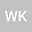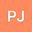Remarks on the infinite-dimensional counterparts of the Darboux theorem
••Wojciech KryszewskiLodz University of Technology Faculty of Technical Physics Information Technology and Applied Mathematics

Corresponding Author:wojciech.kryszewski@p.lodz.pl

Author ProfilePiotr Juszczyk
Lodz University of Technology Faculty of Technical Physics Information Technology and Applied Mathematics
Author Profile## Abstract

The Darboux theorem, one of the fundamental results in analysis, states that the derivative of a real (not necessarily continuously) differentiable function defined on a compact interval has the intermediate value property, i.e. attains each value between the derivatives at the endpoints. The Bolzano intermediate value theorem, which implies Darboux’s theorem when the derivative is continuous, states that a continuous real-valued function $f$ defined on $[-1,1]$ satisfying $f(-1)<0$ and $f(1)>0$ 0, has a zero, i.e. $f(x) = 0$ for at least one number \$-1
13 Jun 2022Submitted to Mathematical Methods in the Applied Sciences
14 Jun 2022Submission Checks Completed
14 Jun 2022Assigned to Editor
20 Jun 2022Reviewer(s) Assigned
09 Sep 2022Review(s) Completed, Editorial Evaluation Pending
26 Sep 2022Editorial Decision: Revise Minor